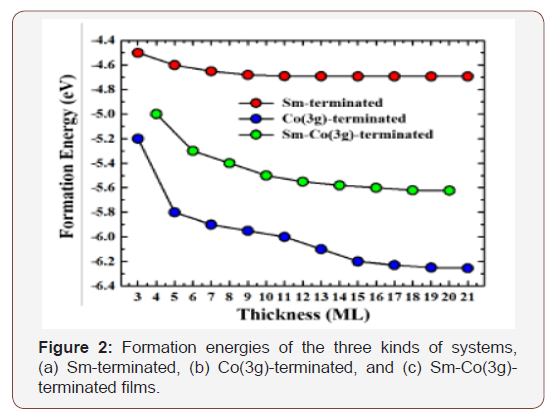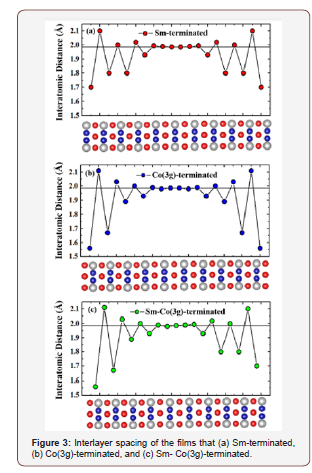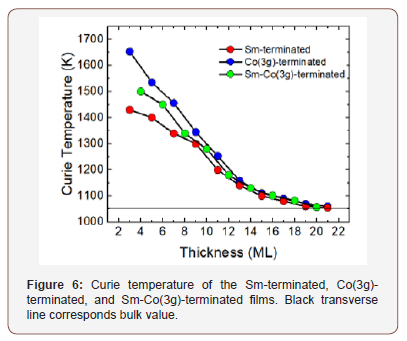# Zero Point Level Effects on Structure, Energy and Spectra of Molecules & Materials

Bruce S Hudson*

Department of Chemistry, Syracuse University, USA

Corresponding Author

Received Date: December 20, 2019;  Published Date: January 07, 2020

#### Introduction

This review encompasses the considerations that must come into play when dealing with molecules or hydrogen bonded crystals where there are two or more equivalent potential energy minima and those cases where isotopic replacement influences the relative energy or spectral properties such as 13C NMR chemical shifts. In such cases the properties of the zero- point level must be investigated. The specific cases discussed are changes in the relative energies of polymorphic crystal structures, the effect of deuterium substitution on the 13C NMR chemical shifts and the hyperfine coupling constant of a radical species involving addition of either muonium or hydrogen atom. We discuss first molecules with double minimum potentials: ammonia, the potentially aromatic  annulene and polyacetylene. Ammonia has minima in its potential energy at equivalent out of plane positions with a maximum at the D3h planar geometry. The vibrational energy levels of the outof- plane bending mode of the ground electronic state of NH3 are shown in the lower part of Figure 1. The pattern of levels in the 1A1 ground state is due to tunneling splitting. The excited electronic state is D3h symmetric at its minimum. Raman transitions excited with 212.8 nm radiation are shown . annulene Figure 2. with N = 4n+2 for n = 4 is potentially aromatic. X-ray diffraction gives D6h symmetry for this material, but this structure does not agree with calculations of proton NMR.High-level calculations of annulene  based on structures selected for agreement with NMR spectra lead to a bond-alternate C2 form over the non-alternating planar D6h structure deduced from diffraction, IR and electronic spectral studies. We have shown that computed Raman spectra for the D6h and C2 forms are expected to be very different, that the zero-point level is above the barrier for this species, and NMR calculations with inclusion of zero-point level averaging were computed by computing the NMR chemical shifts at each geometry, multiplying by the . The green curve in the above is the probability distribution for the structure and the two grey curves are the proton NMR chemical shift values (times 10) for the inside and the outside protons. The resulting averaged values are in reasonable agreement with experimental NMR results . The horizontal red lines are the zero point energy above the vibrational barrier and the first vibrationally excited level of the bond alternation motion which is a normal mode of the molecule.The treatment of the vibrations of polymeric materials is based on the work of Born and van Karman  in which the treatment of the dynamics of a periodic structure starts with a vibrational analysis of the individual repeat unit which is then multiplied by a periodic function whose argument specifies the relative phase shift of the excitations specified by k. Figure 4 from  shows the first step in this procedure. In this case and the two above cases the potential is highly anharmonic and must be treated by numerical methods. Polyacetylene is believed to exhibit bond alternation although there is no direct evidence that this is the case. The difficulty is that the polyacetylene has never been made such that the polymer is in the fully extended all trans all s-trans form. A recent paper  surveys the experimental results and argues that the samples of polyacetylene used in the determination of bond alternation are predominantly comprised of polyenes of finite lengths. These exhibit bond alternation due to end effects. This paper  also introduces a method for the preparation of such a material that forces the polymer chain guest molecules to be constrained to be fully extended and parallel to other chains in a crystalline host lattice. The fully extended all trans polymer in the all s-trans conformation certainly has two equivalent minima in its potential differing by the phase of the bond alternation. However, the zero point energy is, again, well above the barrier [5,6]. The potential energy at each of the solid circular points is obtained from a periodic DFT computation. The solid red lines are the lowest energy anharmonic vibrational levels for this potential for motion of a CH group such the C=C bond expands and the C-C bond contracts. The grey curve is the probability distribution showing that the most probable geometry is above the barrier so that there will be no observable bond alternation. This means that polyacetylene with a fully extended structure will be a metallic conductor. In order to observe this electrical conductivity, it will be necessary that the fully conjugated polyacetylene chains extend from one end of a crystal to another. A method for preparation of such a crystal is under development .The use of isotopic substitution has a long history in molecular spectroscopy. This is especially true of replacement of hydrogen atoms by deuterium, i.e., H- >D. The effect on the 13C NMR chemical shift of replacement of a H by a D for a hydrocarbon with a rigid structure can in principle be computed by consideration of the effect of change in the mean value of the C-H bond length in going from H to D due to stretching anharmonicity times the response in the 13C chemical shift with C-H bond length change. To this must be added the contraction in the CH (CD) bond length stretch and the contraction on going from H to D of the bond angle bending degree of freedom. The change in the 13C chemical shift is largest at the point of substitution but can be measured at more distant points resulting in a lot of comparisons of computation with experiment. The three cases of adamantane, norbornane and protoadamantane are shown below. This results in 83 comparisons of theory with experiment. The magnitude of the effect ranges from over 400 ppb for the point of substitution and ranges down to negative values when odd number rings are present. The RMS deviations for the individual cases are in .

Another example of the reasoning used above in the computation of the isotope effect of H D replacement on 13C chemical shift is the analogous process in which the reactive species C6H7 the cyclohexadiene radical is compared to C6H5Mu where Mu is muonium. Muonium has a mass of 1/9 amu. In this case the local mode calculation of the isotope effect involves computation of the hyperfine coupling constant as a function of the light atom bending and stretching motions and then averaging this variation using the corresponding probability distribution functions obtained from numerical computations of the relevant wavefunctions . In this case there is an extra issue that comparison with experiment requires computation of the value of the hyperfine coupling constant at the equilibrium geometry. This requires high level open shell correlated methods. It is found that several of these methods provide reasonable overall agreement with experiment .Polymorphic crystal pairs provide another area where the zero point and thermal excitation energies involving highly anharmonic potentials results in differences in the relative stability of polymorphic forms. This occurs for glycine  and for the keto and enol tautomeric forms of barbituric acid . What is observed is that the exchange of H by D results in a change in the relative order of stability of the polymorphic forms.

Hydrogen bonds can also have double minimum potentials in crystals including some that are centrosymmetric. The strongest hydrogen bonds involve sharing of a hydrogen by two electronegative heavy atoms, N or O or F. One such case is that of the Zundel cation [H5O2]+ as in perchloric acid dihydrate. [11,12] The centrosymmetric [H5O2]+ cation has a central H and four peripheral H ions weakly hydrogen bonded to perchloric acid anions. The [H2O -H-OH2]+ cation sits at the inversion center. The potential energy for the motion of the central H has a double minimum potential and has a low frequency for vibrational stretching excitation. The peripheral H atoms have high frequency stretching frequency. If this material is prepared with a 4:1 ratio of D:H, i.e. [D2O-H-OD2]+ the H atom is anticipated to be at the center of symmetry.

The vibrational spectroscopy of such material is best investigated using vibrational inelastic neutron scattering as discussed in  and .

#### Acknowledgement

The author would like to acknowledge all of his student and postdoctoral associates especially Larry Ziegler and Damian G. Allis.

#### Conflict of Interest

No conflict of interest.

Article Details
Citation
Keywords
Scroll to Top